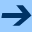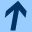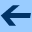On the general solution of the system of cocycle equations without regularity conditions

## On the general solution of the system of cocycle equations without regularity conditions

Jointly written with LUDWIG REICH.

Aequationes mathematicae 68, 200 - 229, 2004.

Abstract: We describe the general solution (α,β), where α=(α(s,x))s∈ ℂ and β=(β(s,x))s∈ ℂ are families of formal power series in C[[x]], of the two so-called cocycle equations

(Co1) α(s+t,x)= α(s,x)α(t,π(s,x)),        s,t∈ ℂ
(Co2) β(s+t,x)= β(s,x)α(t,π(s,x)) +β(t,π(s,x)),         s,t∈ ℂ
together with the boundary condition
(B1) α(0,x)=1,         β(0,x)=0,
where π=(π(s,x))s∈ ℂ is an iteration group in C[[x]]. Our method is based on the knowledge of the regular solutions of (Co1) and (Co2) and on a well-known and often used theorem concerning the algebraic relations between exponential functions and additive functions.
harald.fripertinger "at" uni-graz.at, March 4, 2019